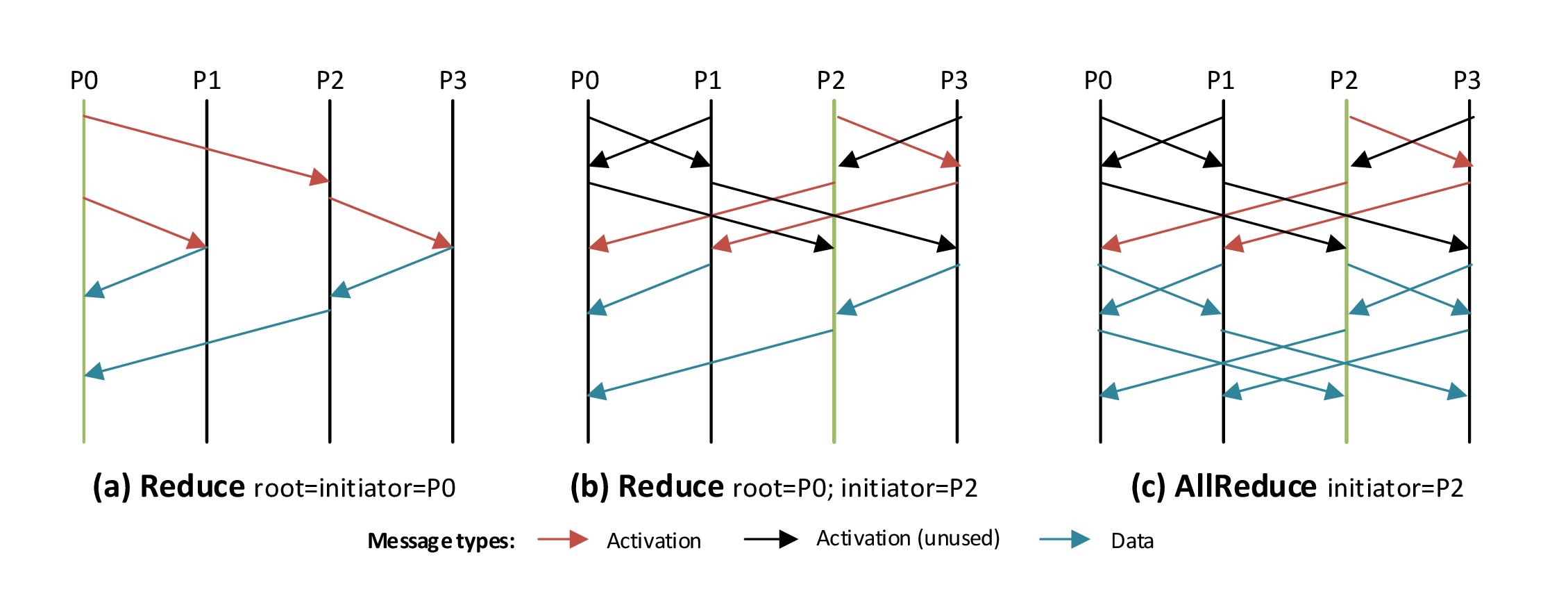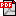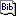SPONSORSCOLLABORATIONS### FFlib - oFFloaded Communication Library

#### Motivation

Network interface cards are one of the key components to achieve efficient parallel performance. In the past, they have gained new functionalities such as lossless transmission and remote direct memory access that are now ubiquitous in high-performance systems. Prototypes of next generation network cards now offer new features that facilitate device programming. FFlib offers a programming interface that allows to express fully offloaded communications/computations, implementing the abstract machine model presented in LINK. The library is built on top of the Portals 4 specification.

#### Abstract Machine Model

This model considers two computational units: the CPU and the Offloading Engine (OE). An operation is defined on the CPU and its execution is fully offloaded to the OE.

In this model all the operations are non-blocking. We define two types of operations: two-sided communications and computations. Happens-before relations can be established among operations; we use the notation $a \rightarrow b$ to indicate that $b$ can be executed only when $a$ is completd. Multiple dependencies can be handled with AND or OR policies. An operation is said CPU-dependent if it can be executed only when explicitely indicated by the CPU.

#### Performance Model

Let $x$ and $y$ be two operations where $x \rightarrow y$, assume that $x$ is a receive operation and it is the only dependency of $y$: once a message matching $x$ is received, $y$ must be executed. In order to make this step, the following sequence of events/ac:Wtions must be handled: receive; matching; execution of $y$. In order to start the execution of $y$ independently from the CPU, this entire sequence must be performed in an offloaded manner. This introduces the requirement that the message matching phase must be performed directly by the OE.

In order to catch this behavior, we extend the well-known LogGP LINK model introducing a new paramenter. called $m$ that models the time needed to: 1) perform the matching phase; 2) satisfy the outgoing dependencies of the matched receive.The above figure illustrates how the model can be applied to a ping-pong communication between the processes P0 and P1. As soon as P0 receives the message from P1 it responds with another message: this means that on P0 the sending of the "pong" message depends on the receive of the "ping". In our model, this dependency is handled and solved directly by the OE, without CPU intervention. The same behavior cannot be modeled in the LogGP model, since in that case we should count an additional $o$ after receiving the "ping" message and before the sending the "pong" one.

#### Library Interface

A send, receive or a computation can be created through the following functions. Each of them returns an operation handle (ff_op_h) that can be used in the subsequent operations.

ff_op_h ff_send(void * buffer, ff_size length, ff_peer peer, ff_tag tag);
ff_op_h ff_recv(void * buffer, ff_size length, ff_peer peer, ff_tag tag);
ff_op_h ff_compute(void * buffer_a, void * buffer_b, ff_size length,
ff_operator operator, ff_datatype datatype, ff_tag tag); 

Once an operation is created, it must be offloaded to the OE in order to be executed.

int ff_post(ff_op_h operation);

The user can wait/test for the completion of a single operation using the following function:

int ff_wait(ff_op_h operation);
int ff_test(ff_op_h operation);

An happens-before relation between two operations can be established with the following function:

int ff_hb(ff_op_h parent, ff_op_h child);

An operation can be subject to multiple dependecies. In this case, the user can specify if the AND or the OR policy must be applied setting the option FF_DEP_AND (default) or FF_DEP_OR, respectively.

int ff_setopt(ff_op_h operation, int options);

#### Collective Communications Offloading

FFlib implements collective communication in a way such that the following two conditions are satisfied:

• No synchronization is required in order to start the collective operation. Every process can start the operation without synchronizing or communicating with the others.
• Once it has started, no further CPU intervention is required. The collective can complete without any CPU intervention.

A collective operation can be defined as a set of schedules, where each node participates to the collective executing its own schedule. A schedule is defined as a local dependency graph where a vertex is an operation, while an edge represents a dependency. It describes a partially ordered set of operations (i.e., point-to-point communications and local computations).

The collective operations currently implemented in FFlib are:

ff_schedule_h ff_barrier(ff_tag_t tag);

ff_schedule_h ff_broadcast(void * data, int count, ff_size_t unitsize, ff_peer_t root, ff_tag_t tag);
ff_schedule_h ff_reduce(void * sndbuff, void * rcvbuff, int count, ff_peer_t root, ff_tag_t tag, ff_operator_t _operator, ff_datatype_t datatype);
ff_schedule_h ff_gather(void * sndbuff, void * rcvbuff, int count, ff_size_t unitsize, ff_peer_t root, ff_tag_t tag);
ff_schedule_h ff_scatter(void * sndbuff, void * rcvbuff, int count, ff_size_t unitsize, ff_peer_t root, ff_tag_t tag);

ff_schedule_h ff_allgather(void * sndbuff, void * recvbuff, int count, ff_size_t unitsize, ff_tag_t tag);
ff_schedule_h ff_allreduce(void * sndbuff, void * recvbuff, int count, ff_tag_t tag, ff_operator_t _operator, ff_datatype_t datatype);
ff_schedule_h ff_alltoall(void * sndbuff, void * recvbuff, int count, ff_size_t unitsize, ff_tag_t tag);


Moreover, the library allows users to create their own schedules (ff_schedule) starting from basic operations (ff_op_h).

#### Solo Collectives

Traditionally, collective communications lead to the pseudo-synchronization of the participating nodes: at the end of the communication all the nodes have reached a point in which the collective call has been started. We refer this semantic as synchronized.

The idea of solo collectives is to globally start the operation as soon as one of the participating nodes (i.e., the initiator) joins the collective, independently from the state of the others. The main consequence of this approach is the relaxation of the usual synchronized semantic.In order to execute a synchronized collective, each process $i$ must create and execute, offloading the execution or not, its schedule $S_i$. Let us define $t=max_i(t_i)$ where $t_i$ is the time at which process $i$ starts the execution of $S_i$. A synchronized collective can be considered as concluded (i.e., all the $S_i$'s have been executed) at a time $\bar{t}_s \ge t$. In a solo collective, each node creates and offloads its schedule $S_i$ at time $k$. At that time the schedule is not executed, but only offloaded to the OE: a schedule in this state is defined as \textit{inactive}. A node that wants to start the collective at time $t_{init}$ activates its own schedule. The activation of a schedule leads to the broadcasting of a control message, necessary to activate the schedule of all the others nodes. A solo collective operation can be considered as concluded at time $\bar{t}_a \ge t_{init} +\epsilon$, where $\epsilon$ is the activation overhead that can be bounded with $\epsilon \le max_i(\epsilon_i)$, with $\epsilon_i$ representing the time required to activate the schedule of node $i$. Please note that, differently from the synchronized case, $\bar{t}_a$ does not depend on any $t_i \neq t_{init}$.

In order to support multiple solo collectives execution we introduce the multi-version schedule (ff_container_h). It allows the pre-posting of $k$ versions of a collective, where a version is a schedule of the same collective, potentially targeting different data buffers. We define three possible states of a posted schedule: disabled, meaning that all the contained operations cannot be executed; enabled, if it is ready to be executed; in use if at least one operation has been completed (e.g., a message is received or sent). A multi-version schedule can be described as a FIFO queue of schedules: at each time there is only one schedule that is enabled. When the enabled schedule becomes in use, then the next in the queue is enabled.

FFlib implements single-source (i.e., broadcast, scatter) and a subset of multiple-source solo collectives. In order to create and execute multiple-source solo collectives the following functions are provided:

ff_schedule_h ff_solo_allgather(void * sndbuff, void * recvbuff, int count, ff_size_t unitsize, ff_tag_t tag);
ff_schedule_h ff_solo_allreduce(void * sndbuff, void * recvbuff, int count, ff_tag_t tag, ff_operator_t _operator, ff_datatype_t datatype);
ff_schedule_h ff_solo_gather(void * sndbuff, void * rcvbuff, int count, ff_size_t unitsize, ff_peer_t root, ff_tag_t tag);


#### Download

 Version Date Changes fflib-0.1.1.tar.gz - (127 KB) June 22, 2015 First release

#### References

 HOTI'15  S. Di Girolamo, P. Jolivet, K. D. Underwood, T. Hoefler: Exploiting Offload Enabled Network Interfaces In Proceedings of the 23rd Annual Symposium on High-Performance Interconnects (HOTI'15), presented in Oracle Santa Clara Campus, CA, USA, IEEE, Aug. 2015, Best Student Paper at HOTI'15